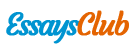# Assignment 2 Fixed Income

Autor:   •  September 7, 2017  •  1,218 Words (5 Pages)  •  363 Views

Page 1 of 5

...

price volatility, assuming that each is trading to offer the same yield to maturity?

Bond

Coupon Rate (%)

Maturity (years)

X

8

9

Y

10

11

Z

11

12

No, we can’t tell from this figures because since both coupon rate and maturity have the effect on the volatility of the price of the bond. The higher of coupon rate will diversify the risk throughout the period so higher coupon rate will decrease the risk while the longer the maturity – the higher the volatility since the bond can’t adjust the term through time change.

5. State why you would agree or disagree with the following statement:

As the duration of a zero-coupon bond is equal to its maturity, the price responsiveness of a zero-coupon bond to yield changes is the same regardless of the level of interest rates.

Disagree because the price responsiveness change given interest rate is in convex relationship. Hence, when the change of interest rate is large, the change in price is larger compare to the price change of interest rate is steep.

10. Consider the following two Treasury securities:

Bond

Price

Modified duration

(years)

A

\$100

6

B

\$80

7

Which bond will have the greater dollar price volatility for a 25-basis-point change in interest rates?

A : dp = -100 * 6 * 0.0025 = -1.5

B: dp = -80 * 7 * 0.0025 = -1.4

So bond B has greater dollar price

17. Consider the following portfolio:

Market Value

Duration (years)

W

\$13 million

2

X

\$27 million

7

Y

\$60 million

8

Z

\$40 million

14

(a) What is the portfolio’s duration?

M.Cap

D

Weight

D*W

13

2

9%

0.19

27

7

19%

1.35

60

8

43%

3.43

40

14

29%

4.00

140

8.96

So, the duration of the portfolio = 8.96. That means when yield changes by 100 bps; the portfolio’s value will change by 8.96% in negative direction.

(b) If interest rates for all maturities change by 50 basis points, what is the approximate percentage change in the value of the portfolio?

Will change by 8.96 * 50 /100 =4.48%

(c) What is the contribution to portfolio duration for each bond?

11. What are the limitations of using duration as a measure of a bond’s price sensitivity to interest-rate changes?

- Measuring sensitivity using duration is accurate only for a small change in yield, there is large error when the interest rate change is high so we use convexity instead. The sensitivity we find still is an approximation.

- To find duration, we have to know cash flow of the asset. For some assets that we can’t the exact number of cash flow we use approximation method.

CHAPTER 5

4. Suppose a client observes the following two benchmark spreads for two bonds:

Bond issue U rated A: 150 basis points

Bond issue V rated BBB: 135 basis points

Your client is confused because he thought the lower-rated bond (bond V) should offer a higher benchmark spread than the higher-rated bond (bond U). Explain why the

benchmark spread may be lower for bond U.

There is more factors than risk rating that determine the spread o the bond. For this situation it could be that Bond U is callable. That is, it gives the issuers the option to call the bond back when they want to. Therefore, it should give higher interest rate than bond V with lower rating since the investor have more risk when the interest rate is down and the Company bought the bond back

Vice versa, Bond V may be putable. That is, it gives the buyers the option to sell the bond back to the firm at a predetermine price during a certain period. Therefore, it should give lower interest rate than bond V with higher rating.

14. You observe the following Treasury yields (all yields are shown on a bond equivalent basis):

Year (Period)

Yield to Maturity (%)

Spot Rate (%)

0.5

...